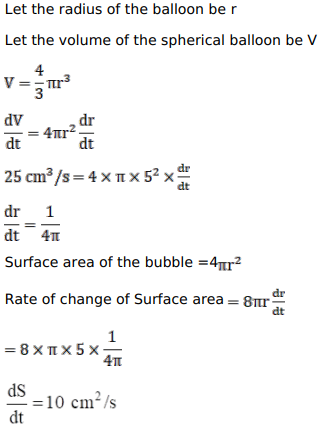# The volume of a spherical balloon is increasing at the rate of 25 cubic centimetres per second.

Question:

The volume of a spherical balloon is increasing at the rate of 25 cubic centimetres per second. Find the rate of change of its surface at the instant when its radius is $5 \mathrm{~cm}$.

Solution: